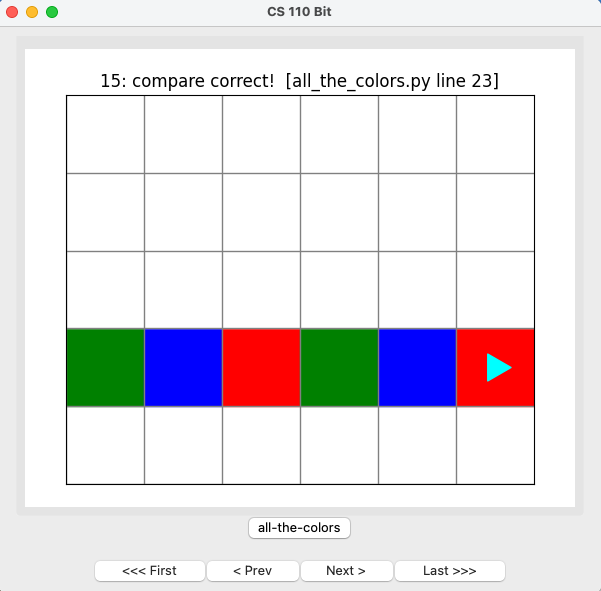Computer Science

# Lab 1a — Introduction to Bit

## Fixing Errors

If you get an error involving byubit or webcolors, open the terminal window in Pycharm. Its icon is found along the left side of the pycharm window, towards the bottom. Copy, paste, and enter

``conda run -n cs110 python -m pip install -U byu_pytest_utils byubit``

If you get an error saying that cs110 is not a conda environment, run this command, then the first command.

``conda create -n cs110 matplotlib pytest``

After creating the conda environment, you will need to tell Pycharm to use it in your project.

## Getting Started

### 5 minutes

#### TAs

Refresh students on how to navigate the website. Show how to get to the lab1a page. Show how labs are submitted in Canvas (Gradescope is only for homeworks).

Ask if there are any students who have not completed lab0 - you may direct them to the TA office if there are several.

#### Students

Download the zip file, shown above. Extract its contents and move the `lab1a` folder into your `cs110` folder. Your folder organization should look like this: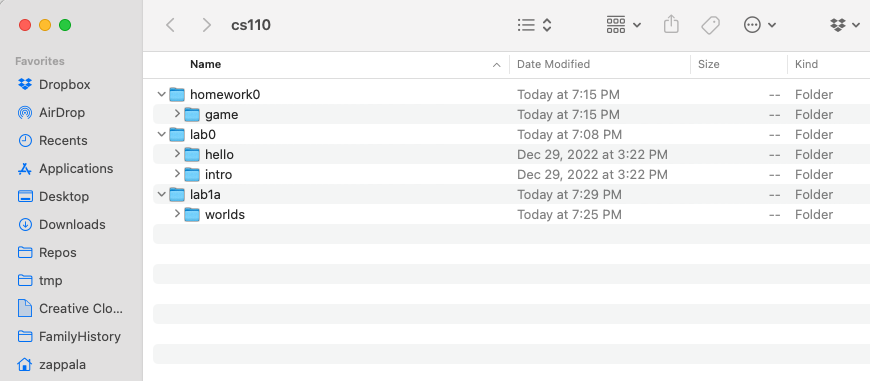Notice that in the `lab1a` folder you have a directory called `worlds`. This directory contains worlds that Bit can operate in. When you see a line like this in a Python file:

``@Bit.worlds('red-dot')``

this tells Bit to use the world called `red-dot.start.txt` inside of the `worlds` folder. It uses `red-dot.finish.txt` to check whether you reached the correct finishing world.

## Exercise 1

### 5 minutes

(a) Can you figure out what this code does?

On a piece of scratch paper, draw out what you think this code will do:

``````from byubit import Bit

@Bit.empty_world(5, 3)
def do_stuff(bit):
bit.paint('blue')
bit.move()
bit.move()
bit.paint('green')
bit.left()
bit.move()
bit.move()
bit.paint('red')

if __name__ == '__main__':
do_stuff(Bit.new_bit)
``````

(b) Run the code

Inside of `lab1a`, create a file called `exercise1.py`. TAs: Show how to create a file several times.

Copy and paste the above code into this file.

Then click on the green triangle next to the main block and run the code:See if your drawing was correct!

If you ran into errors, notify the TA and follow the instructions above.

## Exercise 2

### 5 minutes

(a) Can you figure out what this code does?

On a piece of scratch paper, draw out what you think this code will do:

``````from byubit import Bit

@Bit.empty_world(3, 3)
def do_stuff(bit):
bit.move()
bit.paint('blue')
bit.left()
bit.move()
bit.paint('blue')
bit.move()
bit.left()
bit.move()
bit.paint('blue')
bit.right()
bit.right()
bit.move()
bit.move()
bit.paint('blue')

if __name__ == '__main__':
do_stuff(Bit.new_bit)
``````

(b) Run the code

Inside of `lab1a`, create a file called `exercise2.py`. Copy and paste the above code into this file.

Find the main block and run the code. See if your drawing was correct!

## Exercise 3

### 5 minutes

(a) Can you figure out what this code does?

On a piece of scratch paper, draw out what you think this code will do:

``````from byubit import Bit

@Bit.empty_world(3, 3)
def do_stuff(bit):
bit.paint('blue')
bit.move()
bit.move()
bit.move()
bit.move()
bit.left()
bit.paint('green')
bit.move()
bit.move()
bit.paint('red')

if __name__ == '__main__':
do_stuff(Bit.new_bit)
``````

(b) Run the code

Inside of `lab1a`, create a file called `exercise3.py`. Copy and paste the above code into this file.

Find the main block and run the code. See if your drawing was correct!

## Exercise 4: Red dot

### 10 minutes

You are given some starting code inside of `red_dot.py`: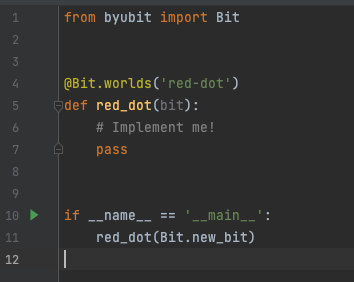Whenever you see a function that has one statement in it — `pass` — this is a function where you need to write code. The `pass` statement is a special keyword in Python that does literally nothing. It just exists as a placeholder for the code you need to write.

For this problem, Bit starts in an empty 3x3 world: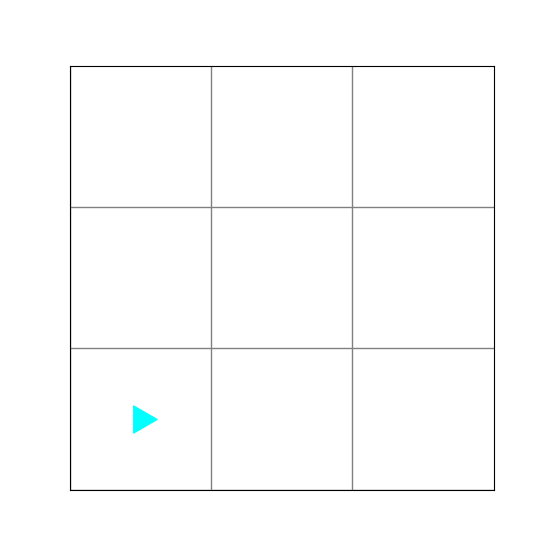Write code in the `red_dot()` function so that the middle square is red. This is what the world should look like when Bit is finished:1. To get started, delete `pass` and write:
``bit.move()``

Be sure the code is indented because in Python indentation matters: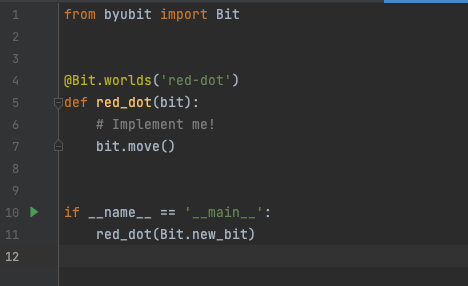Run this code and you will see that you haven’t solved the problem: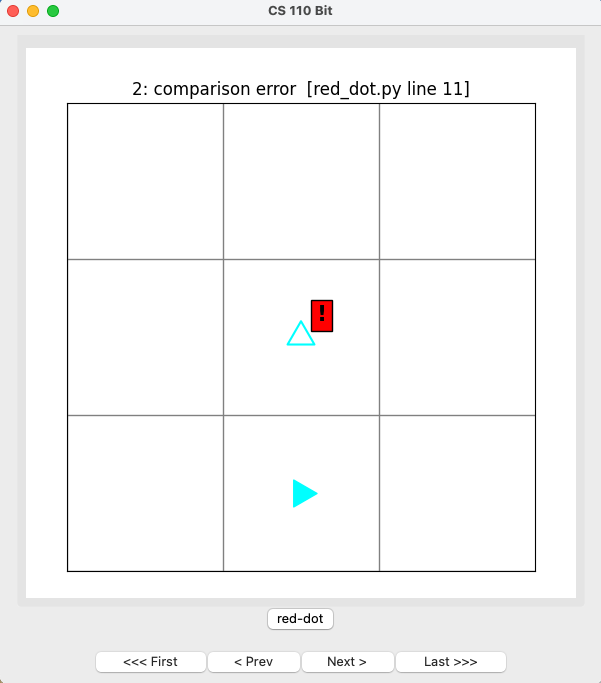Explore the bit window.

• What do you think the exclamation point on the red background means?
• Where is bit currently?
• Where should bit end up?

Now write code to solve this problem.

After writing some code, explore the buttons.

• What does `First` do?
• How would the `Next` button be more useful than Last?
• What do the words at the top of the window mean?
• How do they change as you use the buttons?
• How could you use this information to fix problems in your code?

When you get it right, the screen will tell you `compare correct!` at the top: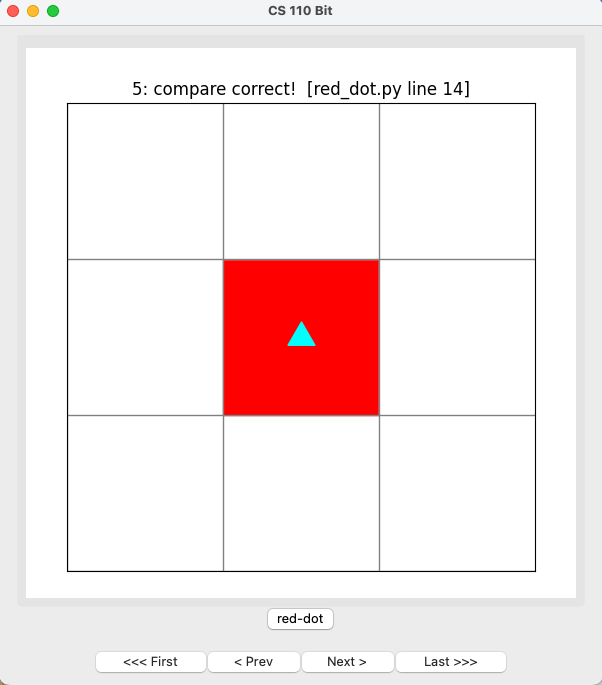## Exercise 5: All the colors

### 10 minutes

You are given some code in `all-the-colors.py`. Bit starts from a blank 5x5 world: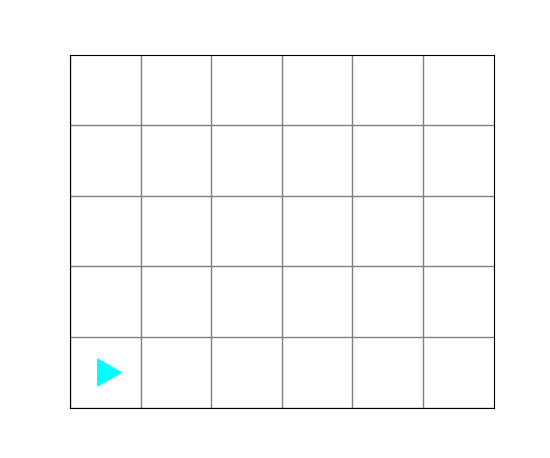Bit should finish with a world that looks like this: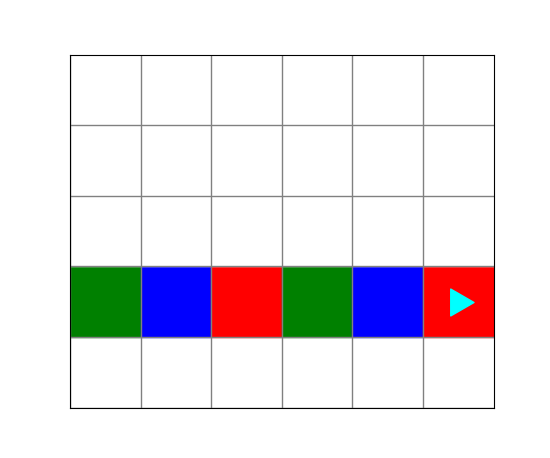Run the code in `all-the-colors.py`. It will finish with a comparison error: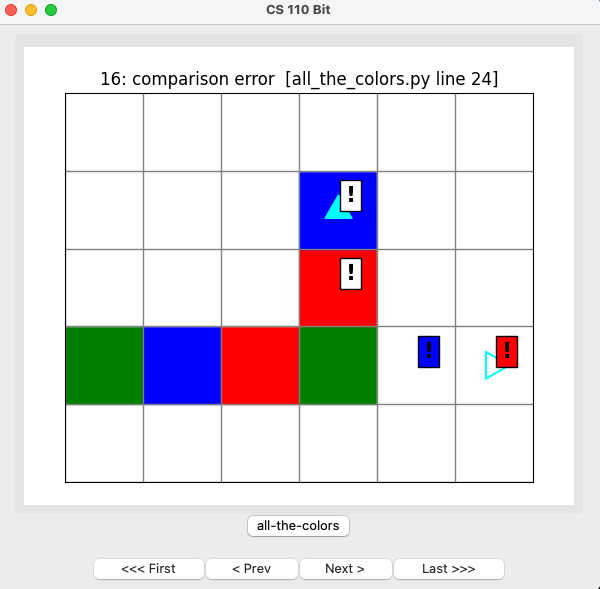• The top two exclamation points have a clear background, showing that these squares should be clear.
• The right two exclamation points have a background that is blue or red, showing that these squares should be blue or red.
• The empty Bit on the right edge shows the location and direction where Bit should be at the end of the problem.

Fix this code so that it works correctly. You should be able to run the code and see that you have it correct: